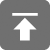# 工学1号馆

home

## 选择排序算法

Wu Yudong    July 25, 2016     Algorithm   497C语言实现：

// Completed on 2014.10.9 08:01
// Language: C99
//
// 版权所有（C）wuyudong
// 博客地址：http://www.wuyudong.com

#include<stdio.h>
#include<stdbool.h>
void swap(int *a, int *b)   //交换两元素的值
{
int t;
t = *a;
*a = *b;
*b = t;
}
void printArray(int a[], int count)   //打印数组元素
{
int i;
for(i = 0; i < count; i++)
printf("%d ",a[i]);
printf("\n");
}
void selection_sort(int *a, int len)
{
register int i, j, min, t;
for(i = 0; i < len - 1; i ++)
{
min = i;
for(j = i + 1; j < len; j ++) //查找最小值
if(a[min] > a[j])
min = j;
if(min != i)           //交换
swap(&a[min], &a[i]);
}
}
int main(void)
{
int a[] = {3, 5, 4, 6, 9, 7, 8, 0, 1};
int n = sizeof(a)/sizeof(*a);
printArray(a, n);
selection_sort(a, n);
printArray(a, n);
return 0;
}